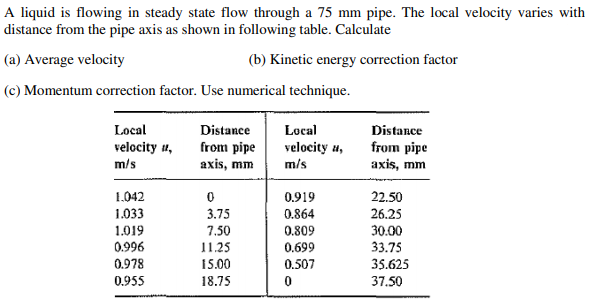# Question A liquid is flowing in steady state flow through a 75 mm pipe. The local velocity varies with distance from the pipe axis as shown in following table. Calculate (a) Average velocity (b) Kinetic energy correction factor (c) Momentum correction factor. Use numerical technique. Local velocity #, m/s Distance from pipe axis, mm Local velocity , m/s Distance from pipe axis, mm 1.042 1.033 1.019 0.996 0.978 0.955 0 3.75 7.50 11.25 15.00 18.75 0.919 0.864 0.809 0.699 0.507 0 22.50 26.25 30.00 33.75 35.625 37.5030QBND The Asker · Mechanical EngineeringTranscribed Image Text: A liquid is flowing in steady state flow through a 75 mm pipe. The local velocity varies with distance from the pipe axis as shown in following table. Calculate (a) Average velocity (b) Kinetic energy correction factor (c) Momentum correction factor. Use numerical technique. Local velocity #, m/s Distance from pipe axis, mm Local velocity , m/s Distance from pipe axis, mm 1.042 1.033 1.019 0.996 0.978 0.955 0 3.75 7.50 11.25 15.00 18.75 0.919 0.864 0.809 0.699 0.507 0 22.50 26.25 30.00 33.75 35.625 37.50
More
Transcribed Image Text: A liquid is flowing in steady state flow through a 75 mm pipe. The local velocity varies with distance from the pipe axis as shown in following table. Calculate (a) Average velocity (b) Kinetic energy correction factor (c) Momentum correction factor. Use numerical technique. Local velocity #, m/s Distance from pipe axis, mm Local velocity , m/s Distance from pipe axis, mm 1.042 1.033 1.019 0.996 0.978 0.955 0 3.75 7.50 11.25 15.00 18.75 0.919 0.864 0.809 0.699 0.507 0 22.50 26.25 30.00 33.75 35.625 37.50